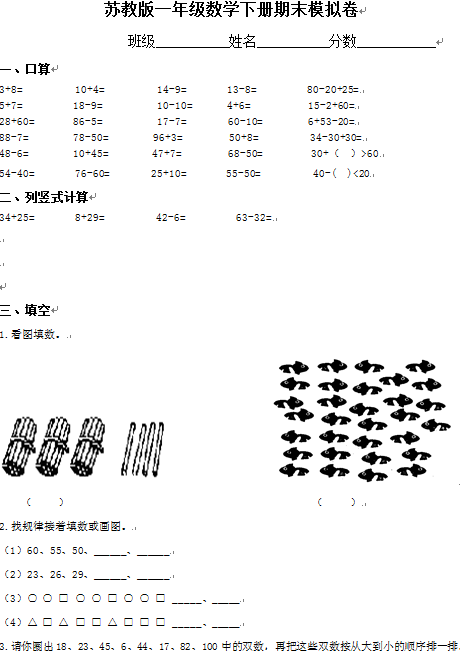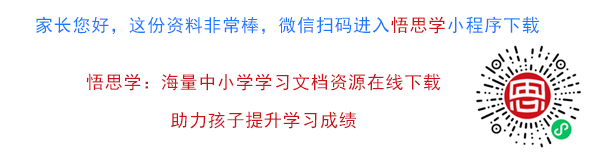3+8=          10+4=          14-9=        13-8=          80-20+25=

5+7=          18-9=           10-10=       4+6=           15-2+60=

【DOC文档31页】【苏教版】一年级下册数学期末考试试卷(9套) A4电子版资料_可直接打印_会员免费下载##### 评论信息